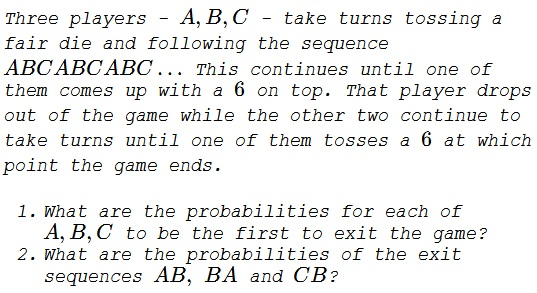# Taking Turns to Toss a Die

### Problem### Solution 1, Question 1

Let $P_A,P_B,P_C$ be the probabilities of $A,B,C,$ respectively, being the first to exit the game. Concerning $P_A,$ when it is $A's$ turn, he either tosses $6$ and the game stops or all three toss anything but $6$ and the game continues:

$\displaystyle P_A=\frac{1}{6}+\left(\frac{5}{6}\right)^3P_A$

from which $\displaystyle P_A=\frac{36}{91}.$ For $B$ to be the first to exit the game, $A$ needs to miss $6$ following by $B$ tossing $6.$ If this does not happen and they (in the $BAC$ sequence) miss $6,$ the game continues:

$\displaystyle P_B=\frac{5}{6}\cdot\frac{1}{6}+\left(\frac{5}{6}\right)^3P_B$

from which $\displaystyle P_B=\frac{30}{91}.$ For $P_C$ we have

$\displaystyle P_C=\left(\frac{5}{6}\right)^2\cdot\frac{1}{6}+\left(\frac{5}{6}\right)^3P_C$

which resolves to $\displaystyle P_C=\frac{25}{91}.$ As a confirmation, $P_A+P_B+P_C=1.$

### Solution 1, Question 2

With $A$ out of the game, the sequence of throws becomes $BCBC\ldots$ If $p_1$ is the probability of $B$ being the first to toss a $6$ then, as before

$\displaystyle P_1=\frac{1}{6}+\left(\frac{5}{6}\right)^2P_1,$

yielding $\displaystyle p_1=\frac{6}{11}.$ Obviously, for $C$ to get ahead of $B,$ the probability $\displaystyle p_2=\frac{5}{11}.$ These probabilities remain valid for a two person sequence, regardless of their identities. Observe that when, e.g., $B$ exits first the game proceeds in the sequence $CACA\ldots$ Thus

\displaystyle\begin{align} P_{AB}&=P_A\cdot p_1=\frac{36}{91}\cdot\frac{6}{11}=\frac{216}{1001}\\ P_{BA}&=P_B\cdot p_2=\frac{30}{91}\cdot\frac{5}{11}=\frac{150}{1001}\\ P_{CA}&=P_C\cdot p_1=\frac{25}{91}\cdot\frac{6}{11}=\frac{150}{1001}\\ P_{CB}&=P_A\cdot p_2=\frac{25}{91}\cdot\frac{5}{11}=\frac{125}{1001}\\ P_{AC}&=P_A\cdot p_2=\frac{36}{91}\cdot\frac{5}{11}=\frac{180}{1001}\\ P_{BC}&=P_A\cdot p_1=\frac{30}{91}\cdot\frac{6}{11}=\frac{180}{1001}. \end{align}

It is easily checked that all six add up to $1,$ as they should. Perhaps surprisingly $P_{BA}=P_{CA}$ and $P_{AC}=P_{BC}.$

### Solution 2

Let $A_k$ be the event that $A$ is the $k^{th}$ person to exit (Similar notation for $B$ and $C$).

\displaystyle \begin{align} P(A_1)&=\frac{1}{6}\left[1+\left(\frac{5}{6}\right)^3+\left(\frac{5}{6}\right)^6+... \right]\\ &=\frac{1/6}{1-(5/6)^3}=\frac{36}{91}, \\ P(B_1)&=\left(\frac{5}{6}\right)P(A_1)=\frac{30}{91}, \\ P(C_1)&=\left(\frac{5}{6}\right)P(B_1)=\frac{25}{91}. \end{align}

\displaystyle \begin{align} P(B_2|A_1)&=P(C_2|B_1)=P(A_2|C_1)\\ &=\frac{1}{6}\left[1+\left(\frac{5}{6}\right)^2+\left(\frac{5}{6}\right)^4+...\right]\\ &=\frac{1/6}{1-(5/6)^2}=\frac{6}{11}, \\ P(C_2|A_1)&=P(A_2|B_1)=P(B_2|C_1)=1-P(B_2|A_1)=\frac{5}{11}. \end{align}

Thus,

\displaystyle \begin{align} P(A_1\cap B_2)&=P(B_2|A_1)P(A_1)=\left(\frac{6}{11}\right)\left(\frac{36}{91}\right)=\frac{216}{1001}, \\ P(A_1\cap C_2)&=P(C_2|A_1)P(A_1)=\left(\frac{5}{11}\right)\left(\frac{36}{91}\right)=\frac{180}{1001}, \\ P(B_1\cap C_2)&=P(C_2|B_1)P(B_1)=\left(\frac{6}{11}\right)\left(\frac{30}{91}\right)=\frac{180}{1001}, \\ P(B_1\cap A_2)&=P(A_2|B_1)P(B_1)=\left(\frac{5}{11}\right)\left(\frac{30}{91}\right)=\frac{150}{1001}, \\ P(C_1\cap A_2)&=P(A_2|C_1)P(C_1)=\left(\frac{6}{11}\right)\left(\frac{25}{91}\right)=\frac{150}{1001}, \\ P(C_1\cap B_2)&=P(B_2|C_1)P(C_1)=\left(\frac{5}{11}\right)\left(\frac{25}{91}\right)=\frac{125}{1001}. \end{align}

### Solution 3

#### Question 1

The probabilities of the event occurring at period $i$: $p (1-p)^{i-1}$ Let $P(.)$ be the probability that the player $A,B$, or $C$ goes tapioca first.

So, skipping 3 for each, we get:

\displaystyle \begin{align} P(A)&=\sum _{i=0}^{\infty } p (1-p)^{3 i}=\frac{1}{(p-3) p+3}=\frac{36}{91},\\ P(B)&=\sum _{i=0}^{\infty } p (1-p)^{3 i+1}=\frac{1-p}{(p-3) p+3}=\frac{30}{91}\\ P(C)&=\sum _{i=0}^{\infty } p (1-p)^{3 i+2}=\frac{(p-1)^2}{(p-3) p+3}=\frac{25}{91} \end{align}

We note that events $A, B,$ and $C$ are separable and exhaustive hence the probabilities sum to unity. This will help in what follows because every combination starting with $A, B,$ or $C$ will entail $P(A)$, $P(B)$, or $P(C)$, respectively.

#### Question 2

Now we condition $AB$ as follows: since we have sequences $BCBCBCBC\ldots$ (after $A)$ and need to skip $2$ for each, starting with $p$.

$\displaystyle P(AB)=P(A)\sum _{i=0}^{\infty } p (1-p)^{2 i}=P(A) \frac{1}{2-p}=\frac{216}{1001}$

We condition $BA$ as follows: since we have for subsequent sequence $ACACACAC\ldots$ (after $B)$ we need to skip $2$ for each, but starting with $p (1-p)$.

$\displaystyle P(BA)=P(B) \sum _{i=0}^{\infty } p (1-p)^{2 i+1}=P(B) \frac{1-p}{2-p}=\frac{150}{1001}$

As to $CB,$ we have subsequent sequence (after the $C)$ $ABABABABABAB\ldots$

$\displaystyle P(CB)=P(C) \sum _{i=0}^{\infty } p (1-p)^{2 i+1}=P(B) \frac{1-p}{2-p}=\frac{125}{1001}$

### Acknowledgment

This based on a problem from P. Nahin's Will You Be Alive 10 Years from Now? (Princeton University Press, 2014, 59-61)

Solution 2 is by Amit Itagi; Solution 3 is by N. N. Taleb.